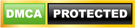Blog

# Defining Functions - Python

• ###### | 1919 Ratings

A function is a block of organized, reusable code that is used to perform a single, related action. Functions help in organizing code. Functions provide better modularity for your application and a high degree of code reusing.

As you already know, Python gives you many built-in functions like print(), etc., but you can also create your own functions. These functions are called user-defined functions.

Learn how to use Python, from beginner basics to advanced techniques, with online video tutorials taught by industry experts. Enroll for Free Python Training Demo!

Python provides some basic user defined functions that are built-in. Programmers can also build their own functions. There are many built in functions in Python and it is not possible to describe all over here. However, the following table explains some of the commonly used built in functions.

 Function Description abs(x) To get the absolute value of number x. all(iterable) If all elements of the iterable are true, then this function will return a true any(iterable) If any element of the iterable is true, then this function will return true basestring() Used to check if an object is a string or a unicode bin(x) Convert integer to a binary string bool(x) Converts a value to boolean –and  returns true or false bytearray(obj) If obj is callable it returns true else it returns false chr(i) For I in the range of 0 to 255 it returns a string of one character whose ASCII code is the integer i cmp(x,y) For objects x and y, the function returns negative integer if x zero if x==0 and positive integer is x>y complex([real[, imag]]) Converts string or a number into a complex number. delattr(object, name) This function helps in deleting an attribute of an object. The object should have a attribute with the name. dict() Creates object of dictionary class divmod(a,b) Where a and b are two non complex numbers, the function will return a pair of numbers that returns quotient and remainder. file(name[, mode[, buffering]]) Constructor function for the file type filter(func, iterable) Construct a list from those elements of iterable for which function returns true Float([x]) Converts x to number of floating point. If x is a string object then it must contain a decimal or a floating point number frozenset([iterable]) Returns frozenset object getattr (object, name) Is same as object.name hasattr (object, name) If name is one of the attributes of the object then it returns true or else false is returned id(object) Returns identity of the object int(n) Convert n to integer len(s) Returns length of objects long(n) Converts n to long max(n) Return largest item of string, tuple or list min(n) Return smallest item of string, tuple or list pow(x,y) Result is x raised to the power of y round(j,k) Floating point value of j is rounded to k digits

For defining your own functions, you will have to remember the following:

1. A function is defined with the help of the keyword def

2. The function name must end with parentheses ‘()’ followed by colon ‘:’ The syntax for defining a function is as follows:

def function_name(args):

code_to_execute

Functions are completely independent of the main program. You may consider it as a mini independent program that may require some parameters for execution. The main program will only about the value returned by the function, and it is not concerned about what is actually done.

-Once a function has been designed it can be called from anywhere in the program

Format:

`function_name(args)`

EXAMPLE

```def whoAmI(str):
print ‘I am’,str
a=’Apple’
b=7
c =’Cat’
whoAmI(a)
whoAmI(b)
whoAmI(c)```

Output: I am Apple; I am 7; I am Cat

-Making a function return a value

Format:

```def function_name():

code_to execute

return value```

EXAMPLE

```def whoAmI(str):
return str
a=’Apple’
b=’ball’
c =’Cat’
print whoAmI(a)
print whoAmI(b)
print whoAmI(c)```

output: I am Apple; I am ball; I am Cat

HTTPS://DOCS.PYTHON.ORG/RELEASE/1.5.1P1/TUT/FUNCTIONS.HTML